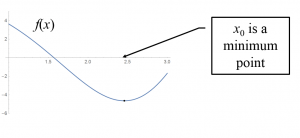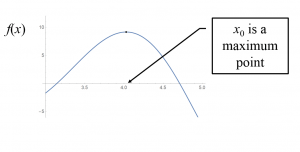15
Sep 17

## Geometry behind optimization## Geometry behind optimization

Optimization is an animal of many faces. More precisely, this post is about what is called the First Order Conditions (FOC's) and Second Order Conditions (SOC's). Make sure to go through this visual introduction to derivatives, then everything will be easy.

## Case of a minimum

Draw a simple function that has a clear minimum. For example, just use a parabola with branches looking upward. Below it draw the graph of its derivative. Satisfy yourself that the derivative at the minimum point is zero. Then look at the behavior of the second derivative at the minimum point. Establish its sign; that will be the second order condition.Video 1. Conditions sufficient for a minimum point

## Case of a maximum

Here you turn the previous discussion upside down. Draw a simple function that has a clear maximum. For example, just use a parabola with branches looking downward. Below it draw the graph of its derivative. Satisfy yourself that the derivative at the maximum point is zero. Then look at the behavior of the second derivative at the maximum point. Establish its sign; that will be the second order condition.Video 2. Conditions sufficient for a maximum point

## When first and second order conditions don't work

The example in case is the cubic function. The derivative at zero is zero. However, the origin is neither maximum nor minimum. This is an inflection point (concavity changes to convexity). The general rule that arises from the analysis of the Taylor decomposition is this. Look for the first derivative in the decomposition that is not zero. If the order of the derivative is even, then the sign of the derivative determines whether it's a maximum or a minimum. If the order of the derivative is odd, then it's an inflection point, regardless of the sign of the derivative.

## Summary

A point where the first derivative vanishes is called a critical point. Suppose$x_0$ is a critical point.

Case 1. If the second derivative at$x_0$ is positive, you have a minimum because locally the function is a parabola with branches extended upward.

Case 2. If the second derivative at$x_0$ is negative, you have a maximum because locally the function is a parabola with branches hanging downward.

Case 3. If the second derivative at$x_0$ is zero, you are out of luck. Look for the first derivative$f^{(n)}$ that is different from zero at$x_0$. Your course of action is explained by the fact that locally the function is$f(x)\approx f(x_0)+\frac{f^{(n)}}{n!}(x-x_0)^n.$

If$n$ is even, it is a minimum in case$f^{(n)}(x_0)>0$ and a maximum in case$f^{(n)}(x_0)<0$. If$n$ is odd, it's neither, and the investigation is over.